# OOF: Finite Element Analysis of Microstructures

/equilibrateNext: /comp_equil Up: The Main OOF Menu Previous: /commandfile   Contents

## /equilibrate

Find the solution for the current boundary conditions, distortion and temperature.

The solver uses an iterative sparse matrix method.3.2 The number of iterations which the solver will attempt is fixed by the variable maxiter. If convergence to a solution is not achieved by maxiter iterations, then equilibrate can be re-invoked to start from the current state.

When solving the matrix equation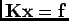, the solution is considered to be converged when the norm of the residual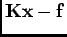is less than the variable tolerance times the norm of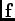. f is the vector of external and thermal forces, K is the stiffness matrix, and the vector x is the degrees of freedom being solved for.

The solution method can be adjusted by the variable preconditioner and the boolean variable globalK. The preconditioner is a matrix M which multiplies both sides of. A good preconditioner nearly diagonalizes K and hence speeds up the iterative solution. The iterative method does not actually require that the matrix K be constructed and stored as a global matrix--only that its individual components can be computed when needed. However, some of the preconditioners require the global matrix. Set variable globalK to true if you want the global matrix to be computed. The choices for preconditioners are

none
Use no preconditioner (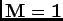).

diagonal
M is the inverse of the diagonal elements of K. This works well only if K is strongly diagonally dominant, but the preconditioning step is fast and simple.

block
This is the same as diagonal, but M is the inverse of the block diagonal elements of K, where each block corresponds to the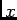and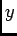degrees of freedom of one node in the mesh. This doesn't work as well as we'd hoped, but it's still fast to implement.

ILU
Incomplete LU decomposition. This seems to work the best, but requires globalK = true.

ICP
Incomplete Cholesky decomposition. Also requires globalK = true.Next: /comp_equil Up: The Main OOF Menu Previous: /commandfile   Contents
/* Send mail to the OOF Team *//* Go to the OOF Home Page */